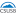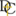Mathematics Commons™

17,858 Full-Text Articles 17,112 Authors 4,137,002 Downloads285 Institutions

All Articles in Mathematics

17,858 full-text articles. Page 4 of 528.

Non-Nested Monte Carlo Dual Bounds For Multi-Exercisable Options, 2019Centre for Actuarial Studies, Department of Economics, The University of Melbourne, VIC 3010, Australia

Non-Nested Monte Carlo Dual Bounds For Multi-Exercisable Options, Xiang Cheng, Zhuo Jin

Communications on Stochastic Analysis

No abstract provided.

Hybrid Models And Switching Control With Constraints, 2019Wayne State University

Hybrid Models And Switching Control With Constraints, Jose L. Menaldi, Maurice Robin

Communications on Stochastic Analysis

No abstract provided.

Totalitarian Random Tug-Of-War Games In Graphs, 2019Universitat Politècnica de Catalunya, Departament de Matemàtiques, Diagonal 647, 08028 Barcelona, Spain

Totalitarian Random Tug-Of-War Games In Graphs, Marcos Antón, Fernando Charro, Peiyong Wang

Communications on Stochastic Analysis

No abstract provided.

201918 Thoroughbred Drive, Sherborn, MA 01770, USA

Stochastic Process And Its Role In The Development Of The Financial Market: Celebrating Professor Chow's Long And Successful Career, Xisuo L. Liu

Communications on Stochastic Analysis

No abstract provided.

Preface, 2019Louisiana State University, Baton Rouge, LA 70803 USA

Preface, Hui-Hsiung Kuo, George Yin

Communications on Stochastic Analysis

No abstract provided.

Subdifferentials Of Value Functions In Nonconvex Dynamic Programming For Nonstationary Stochastic Processes, 2019Department of Mathematics, Wayne State University, Detroit, MI 48202, USA

Subdifferentials Of Value Functions In Nonconvex Dynamic Programming For Nonstationary Stochastic Processes, Boris S. Mordukhovich, Nobusumi Sagara

Communications on Stochastic Analysis

No abstract provided.

Euler-Maruyama Method For Regime Switching Stochastic Differential Equations With Hölder Coefficients, 2019Department of Applied Mathematics, Faculty of Applied Science, HCMC University of Technology, Vietnam

Euler-Maruyama Method For Regime Switching Stochastic Differential Equations With Hölder Coefficients, Dung T. Nguyen, Son L. Nguyen

Communications on Stochastic Analysis

No abstract provided.

Algebraic Methods For Proving Geometric Theorems, 2019California State University, San Bernardino

Algebraic Methods For Proving Geometric Theorems, Lynn Redman

Electronic Theses, Projects, and Dissertations

Algebraic geometry is the study of systems of polynomial equations in one or more variables. Thinking of polynomials as functions reveals a close connection between affine varieties, which are geometric structures, and ideals, which are algebraic objects. An affine variety is a collection of tuples that represents the solutions to a system of equations. An ideal is a special subset of a ring and is what provides the tools to prove geometric theorems algebraically. In this thesis, we establish that a variety depends on the ideal generated by its defining equations. The ability to change the basis of an ideal ...

2019Georgia Southern University

Colored Complete Hypergraphs Containing No Rainbow Berge Triangles, Colton Magnant

Theory and Applications of Graphs

The study of graph Ramsey numbers within restricted colorings, in particular forbidding a rainbow triangle, has recently been blossoming under the name Gallai-Ramsey numbers. In this work, we extend the main structural tool from rainbow triangle free colorings of complete graphs to rainbow Berge triangle free colorings of hypergraphs. In doing so, some other concepts and results are also translated from graphs to hypergraphs.

The Fundamental Theorem Of Classical Mechanics, 2019University of North Georgia

The Fundamental Theorem Of Classical Mechanics, Piotr W. Hebda, Beata Hebda

Faculty Publications

Assuming two given time-independent Newtonian systems of the same dimensions, each of the two systems including its own given set of time-independent generalized Poisson Brackets and a time-independent Hamiltonian, there always locally exists a one-to-one function from variables of one system to the variables of the other system, such that it transforms equations of motion of the first system into equations of motion of the second system, the Poisson Brackets of the first system into the Poisson Brackets of the second system, and the Hamiltonian of the first system into the Hamiltonian of the second system.

One interpretation of the ...

Spn Graphs, 2019Iowa State University

Spn Graphs, Leslie Hogben, Naomi Shaked-Monderer

Electronic Journal of Linear Algebra

A simple graph G is an SPN graph if every copositive matrix having graph G is the sum of a positive semidefinite and nonnegative matrix. SPN graphs were introduced in [N. Shaked-Monderer. SPN graphs: When copositive = SPN. Linear Algebra Appl., 509:82{113, 2016.], where it was conjectured that the complete subdivision graph of K4 is an SPN graph. This conjecture is disproved, which in conjunction with results in the Shaked-Monderer paper show that a subdivision of K_4 is a SPN graph if and only if at most one edge is subdivided. It is conjectured that a graph is an ...

Alpha Adjacency: A Generalization Of Adjacency Matrices, 2019Washington State University

Alpha Adjacency: A Generalization Of Adjacency Matrices, Matt Hudelson, Judi Mcdonald, Enzo Wendler

Electronic Journal of Linear Algebra

B. Shader and W. So introduced the idea of the skew adjacency matrix. Their idea was to give an orientation to a simple undirected graph G from which a skew adjacency matrix S(G) is created. The -adjacency matrix extends this idea to an arbitrary field F. To study the underlying undirected graph, the average -characteristic polynomial can be created by averaging the characteristic polynomials over all the possible orientations. In particular, a Harary-Sachs theorem for the average-characteristic polynomial is derived and used to determine a few features of the graph from the average-characteristic polynomial.

Maps Preserving Norms Of Generalized Weighted Quasi-Arithmetic Means Of Invertible Positive Operators, 2019Institute of Mathematics, University of Debrecen, H-4002 Debrecen, P.O. Box 400

Maps Preserving Norms Of Generalized Weighted Quasi-Arithmetic Means Of Invertible Positive Operators, Gergő Nagy, Patricia Szokol

Electronic Journal of Linear Algebra

In this paper, the problem of describing the structure of transformations leaving norms of generalized weighted quasi-arithmetic means of invertible positive operators invariant is discussed. In a former result of the authors, this problem was solved for weighted quasi-arithmetic means, and here the corresponding result is generalized by establishing its solution under certain mild conditions. It is proved that in a quite general setting, generalized weighted quasi-arithmetic means on self-adjoint operators are not monotone in their variables which is an interesting property. Moreover, the relation of these means with the Kubo-Ando means is investigated and it is shown that the ...

On Sign Pattern Matrices That Allow Or Require Algebraic Positivity, 2019University of the Philippines Diliman

On Sign Pattern Matrices That Allow Or Require Algebraic Positivity, Diane Christine Pelejo, Jean Leonardo Abagat

Electronic Journal of Linear Algebra

A square matrix M with real entries is algebraically positive (AP) if there exists a real polynomial p such that all entries of the matrix p(M) are positive. A square sign pattern matrix S allows algebraic positivity if there is an algebraically positive matrix M whose sign pattern is S. On the other hand, S requires algebraic positivity if matrix M, having sign pattern S, is algebraically positive. Motivated by open problems raised in a work of Kirkland, Qiao, and Zhan (2016) on AP matrices, all nonequivalent irreducible 3 by 3 sign pattern matrices are listed and classify into ...

A Geometric Generalizaion Of The Planar Gale-Nikaidô Theorem, 2019Trinity University

A Geometric Generalizaion Of The Planar Gale-Nikaidô Theorem, Eduardo C. Balreira

Eduardo Cabral Balreira

The Gale-Nikaidô Theorem establishes global injectivity of maps defined over rectangular regions provided the Jacobian matrix is a P-matrix. We provide a purely geometric generalization of this result in the plane by showing that if the image of each edge of the rectangular domain is realized as a graph of a function over the appropriate axis, then the map is injective. We also show that the hypothesis that the Jacobian matrix is a P-matrix is simply one way to analytically check this geometric condition.

Global Stability Of Higher Dimensional Monotone Maps, 2019Trinity University

Global Stability Of Higher Dimensional Monotone Maps, Eduardo C. Balreira, Saber Elaydi, Rafael Luis

Eduardo Cabral Balreira

We develop a notion of monotonicity for maps defined on Euclidean spaces Rk+, of arbitrary dimension k. This is a geometric approach that extends the classical notion of planar monotone maps or planar competitive difference equations. For planar maps, we show that our notion and the classical notion of monotonicity are equivalent. In higher dimensions, we establish certain verifiable conditions under which Kolmogorov monotone maps on Rk+ have a globally asymptotically stable fixed point. We apply our results to two competition population models, the Leslie–Gower and the Ricker models of two- and three-species. It is shown that ...

2019Dordt University

The History Of Mathematics: A Source-Based Approach, Volume 1 (Book Review), Calvin Jongsma

Faculty Work Comprehensive List

Reviewed Title: The History of Mathematics: A Source-based Approach, Volume 1 by June Barrow-Green, Jeremy Gray, and Robin Wilson. Providence : American Mathematical Society, 2019. 488 pp. ISBN: 9781470443528.

The Inverse Eigenvalue Problem For Leslie Matrices, 2019University of Roma "La Sapienza"

The Inverse Eigenvalue Problem For Leslie Matrices, Luca Benvenuti

Electronic Journal of Linear Algebra

The Nonnegative Inverse Eigenvalue Problem (NIEP) is the problem of determining necessary and sufficient conditions for a list of $n$ complex numbers to be the spectrum of an entry--wise nonnegative matrix of dimension $n$. This is a very difficult and long standing problem and has been solved only for $n\leq 4$. In this paper, the NIEP for a particular class of nonnegative matrices, namely Leslie matrices, is considered. Leslie matrices are nonnegative matrices, with a special zero--pattern, arising in the Leslie model, one of the best known and widely used models to describe the growth of populations. The lists ...

On Orthogonal Matrices With Zero Diagonal, 2019Grenfell Campus, Memorial University of Newfoundland

On Orthogonal Matrices With Zero Diagonal, Robert F. Bailey, Robert Craigen

Electronic Journal of Linear Algebra

This paper considers real orthogonal $n\times n$ matrices whose diagonal entries are zero and off-diagonal entries nonzero, which are referred to as $\OMZD(n)$. It is shown that there exists an $\OMZD(n)$ if and only if $n\neq 1,\ 3$, and that a symmetric $\OMZD(n)$ exists if and only if $n$ is even and $n\neq 4$. Also, a construction of $\OMZD(n)$ obtained from doubly regular tournaments is given. Finally, the results are applied to determine the minimum number of distinct eigenvalues of matrices associated with some families of graphs, and the related notion of orthogonal ...

On The Block Structure And Frobenius Normal Form Of Powers Of Matrices, 2019Washington State University

On The Block Structure And Frobenius Normal Form Of Powers Of Matrices, Mashael M. Al Baidani, Judi J. Mcdonald

Electronic Journal of Linear Algebra

The Frobenius normal form of a matrix is an important tool in analyzing its properties. When a matrix is powered up, the Frobenius normal form of the original matrix and that of its powers need not be the same. In this article, conditions on a matrix $A$ and the power $q$ are provided so that for any invertible matrix $S$, if $S^{-1}A^qS$ is block upper triangular, then so is $S^{-1}AS$ when partitioned conformably. The result is established for general matrices over any field. It is also observed that the contributions of the index of cyclicity ...